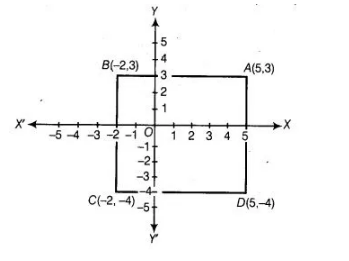# Points A(5, 3), B(-2, 3) and 0(5, – 4) are three vertices of a square ABCD.`
Question:

Points A(5, 3), B(-2, 3) and 0(5, – 4) are three vertices of a square ABCD. Plot these points on a graph paper and hence, find the coordinates of the vertex C.
Thinking Process

(i) Firstly, plot the given points on a graph and join in order.

(ii) Now, we extend a line from point D parallel to X-axis and extend an other line from point 8 parallel to Y-axis, which will meet at point C.

(iii) Further, we measure the distance from point C to the coordinate axis.

Solution:

The graph obtained by plotting the points A, B and C andD is given below. Take a point C on the graph such that ABCD is a square i.e., all sides AB,

BC, CD, and AD are equal.

So, abscissa of C should be equal to abscissa of B i.e., -2 and ordinate of C should be equal to ordinate of D i.e., -4. Hence, the coordinates of C

are (-2, – 4).